# How to Convert Date & Time Format to Date in Excel

Sometimes we want to convert date and time format to date only format in excel for example convert `01/29/2019 06:51:03` to `01/29/2019`, we can convert format by Formula or Format Settings. The two ways are easy to learn, so you can select one you like to convert date and time format.

## Convert Date & Time to Date by Formula

Prepare a table with date and time, the format is `MM/DD/YYYY HH/MM/SS`.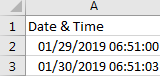If we want to convert them to date format, we can follow below steps:

Step 1: In B2, enter the formula =MONTH(A2) & “/” & DAY(A2) & “/” & YEAR(A2).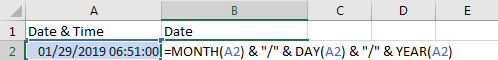Step 2: Click Enter to get the result. Verify that format is changed to date properly, time part is cleared.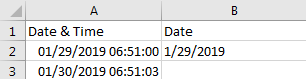Step 3: Drag the Fill Handle down to fill B3. Verify that B3 is changed to date format properly.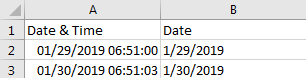## Convert Date & Time to Date by Format Settings

Step 1: On A2, right click to load menu, select Format Cells.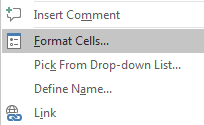Step 2: In Format Cells window, under Number tab, in Category list, select Date; then in Type list select one format you want to show the date. Then the date format is displayed in Sample field.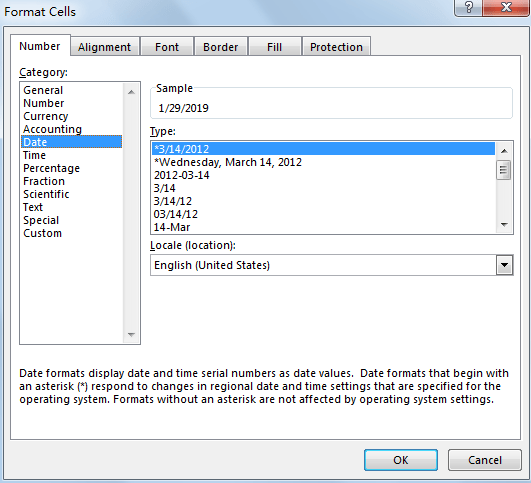Step 3: Click OK. Verify that previous date and time is changed to date format.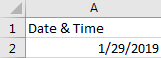### Related Functions

• Excel YEAR function
The Excel YEAR function returns a four-digit year from a given date value, the year is returned as an integer ranging from 1900 to 9999. The syntax of the YEAR function is as below:=YEAR (serial_number)…
• Excel MONTH function
The Excel MONTH function returns the month of a date represented by a serial number. And the month is an integer number from 1 to 12. The syntax of the MONTH function is as below:=MONTH (serial_number)…
• Excel DAY function
The Excel DAY function returns a day of a date (from 1 to 31).The DAY function is a build-in function in Microsoft Excel and it is categorized as a DATE and TIME Function.The syntax of the DAY function is as below:= DAY (date_value)…